# Isosceles trapezium

Trapezoid YSED (YS||ED) is isosceles.
The size of the angle at vertex Y is 17 degrees.
Calculate the size of the angle at vertex E.

Result

angle DES:  163 °

#### Solution:

$\angle DES = 180^\circ - 17^\circ = 163^\circ$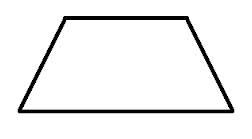Our examples were largely sent or created by pupils and students themselves. Therefore, we would be pleased if you could send us any errors you found, spelling mistakes, or rephasing the example. Thank you!

Leave us a comment of this math problem and its solution (i.e. if it is still somewhat unclear...):Be the first to comment!## Next similar math problems:

1. Rectangular trapezoid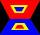How many inner right angles has a rectangular trapezoid?
2. Minute angle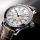Determine size of angle, which takes minute hand for 75 minutes.
3. Angles 1It is true neighboring angles have not common arm?
4. Rhombus anglesIf one angle in the rhombus is 159°, what is it neighboring angle in rhombus?
5. Parallelogram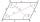In the parallelogram we know one internal angle 67°33`. Calculate the other internal angles.
6. Obtuse angleWhich obtuse angle is creating clocks at 17:00?
7. ZdeněkZdeněk picked up 15 l of water from a 100-liter full-water barrel. Write a fraction of what part of Zdeněk's water he picked.
8. Tenths digit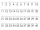For 10.932, which digit is in the tenths place?
9. SimplifySimplify the following problem and express as a decimal: 5.68-[5-(2.69+5.65-3.89) /0.5]
10. LineCan we construct a line segment, if we know: center
11. Simple equation 1035= 7*3*x what is x?
12. In fractionsAn ant climbs 2/5 of the pole on the first hour and climbs 1/4 of the pole on the next hour. What part of the pole does the ant climb in two hours?
13. Write decimalsWrite in the decimal system the short and advanced form of these numbers: a) four thousand seventy-nine b) five hundred and one thousand six hundred and ten c) nine million twenty-six
14. Bus 14Boatesville is 65.35 kilometers from Stanton. A bus traveling from Stanton is 24.13 kilometers from Boatesville. How far has the bus traveled?
15. Simple equation 8Solve the following equation: 36=-(1+7x)-6(-7-x)
16. ExpressionSolve for a specified variable: P=a+4b+3c, for a
17. Fraction and a decimalWrite as a fraction and a decimal. One and two plus three and five hundredths